We will now start a seven-part series that will discuss each Platonic solid in detail as well as associated Archimedean and Catalan solids.

We will begin here with the “master solid”, the tetrahedron.

Freddy Silva writes, “One of the most important alchemical and Hermetic symbols of all time is the tetrahedron (the four-sided pyramid), principally because of its function as the prime bonding pattern of matter.”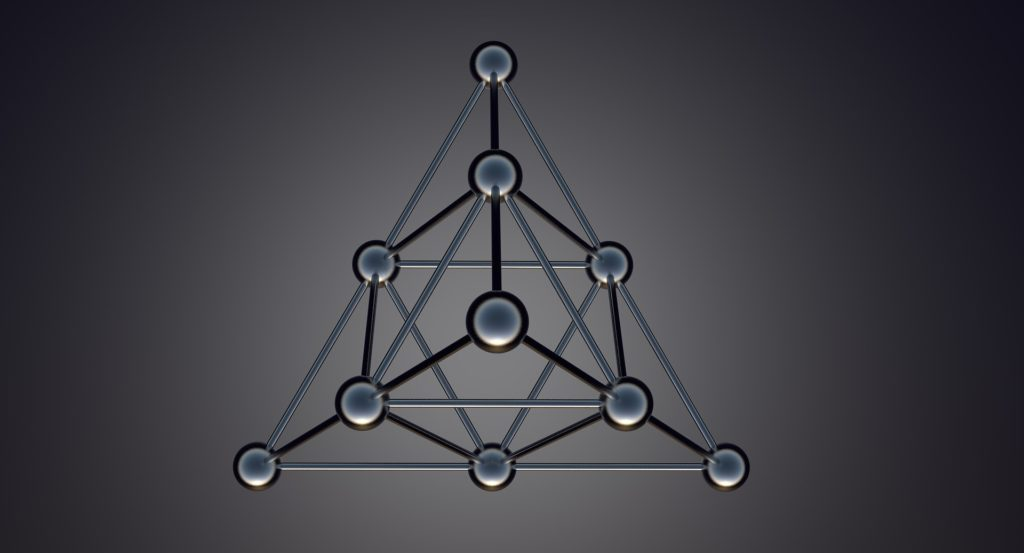Rotating Tetrahedron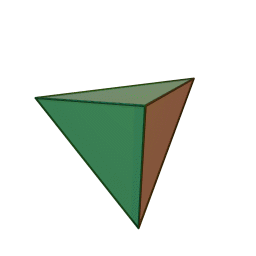Triangle to Tetrahedron

The tetrahedron blossoms up from the flat triangle through the Vesica Piscis.

Michael Schneider writes, “The birth of the tetrahedron, the first volume, brings the Triad’s principle of triangular stability to three dimensions using the fewest materials.”

The 3D Triangle – The Tetrahedron

As we saw in earlier articles, the Vesica Piscis naturally generates the equilateral triangle.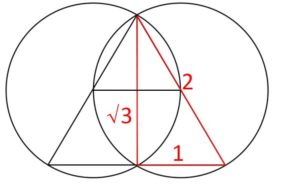The equilateral triangle naturally generates the tetrahedron.

The triangle can be naturally divided into 4 smaller equilateral triangles.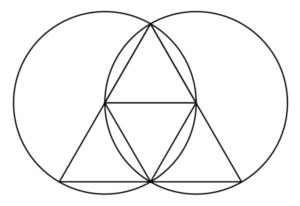These triangles fold inwards towards each other to form the tetrahedron, the first, simplest and most fundamental Platonic solid.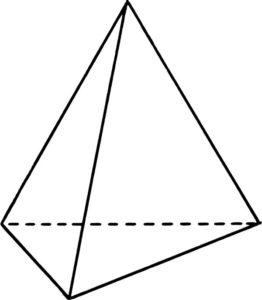To Build a Tetrahedron:

• Construct a Vesica Piscis and large triangle.
• Subdivide the triangle into four smaller triangles by connecting the centers and their intersections.
• Fold the three corners upwards until they meet at one point.
• Attach together to create a tetrahedron.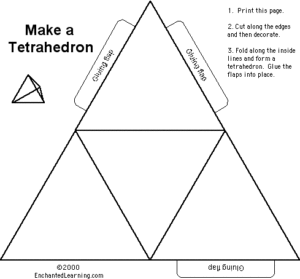The Tetrahedron Net

It was artist Albrecht Durer (1471-1528) to first publish the nets of polyhedra.

“[Durer] introduces a technique of conveying information about three-dimensional objects on a flat surface via paper-folding which in modern times is called a net.  The method involves developing the surface of a polyhedron onto a plane sheet of paper so that the resulting figure can be cut out as a single connected piece then folded up to form a three-dimensional model of the original polyhedron.”1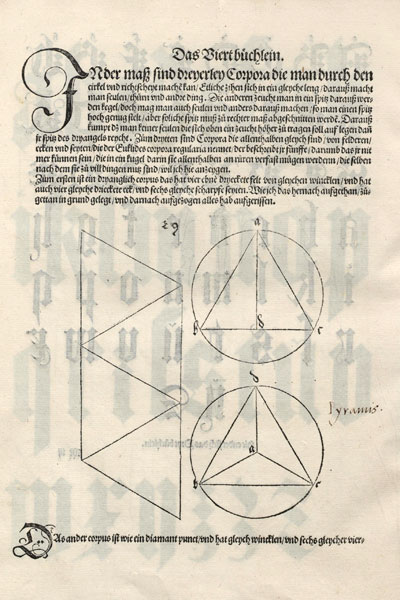Durer’s net was different than the above net.  However they both involve four equilateral triangles.

The Tetrahedron (“four faces”) – 720º

The tetrahedron is the only 3D shape whose corners are the same distance from each other.

As we saw, it consists of four equilateral triangles, with three meeting at each vertex.It has:

• 4 equilateral triangular faces
• 4 vertices
• 6 edges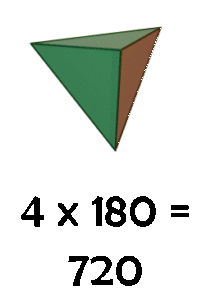It is related to the element ‘Fire’ by Plato due to the penetrating acuteness of its edges and vertices and because it is the simplest and most fundamental of the regular solids.

Robin Waterfield describes it thus, “Fire burns because its particles have sharp edges and are good at cutting, and they also move quickly as they are small.  Hence the phenomenon of burning is due to the cutting action of sharp, rapidly moving particles.”

Furthermore, Fire is nothing more than photons being released in a chaotic, incoherent way from matter.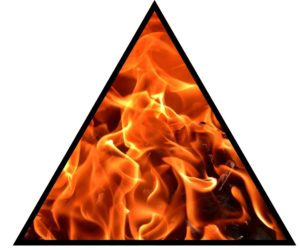So on a deeper symbolic level, the tetrahedron is also called ‘fire’ because it is the shape of the photon or light.

As Plato writes in Timaeus, “Fire is required for the creation of anything visible.”

Freddy Silva adds, “The tetrahedron is a guide to understanding the universal mechanics of light, sound, and magnetism, and how an understanding of such transforms the base metal (physical human) into gold (spiritually enlightened human).”

The tetrahedron is considered by Buckminster Fuller and Nassim Haramein, among others, to be the basic unit of space.

It is the most spare shape.

It encloses the least volume with the greatest relative surface area.  This is opposite of the sphere that encloses the most volume with the least relative surface area.

It is the strongest and most stable solid.

It is impervious to ‘dimpling’.  “Push hard on any vertex and either the whole system turns inside out (if the tetrahedron is made of rubber) or nothing happens; structural resistance prevails.”2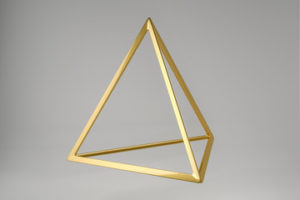Its dual is an inverse tetrahedron.  Together they create the star tetrahedron, otherwise known as the stellated octahedron, or stella octangula.

Four spheres stack as a tetrahedron: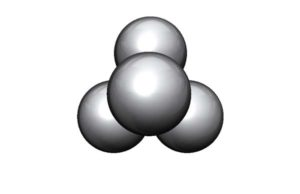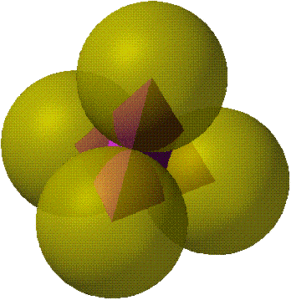The spherical tetrahedron: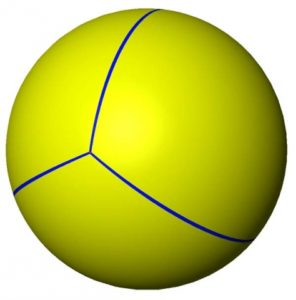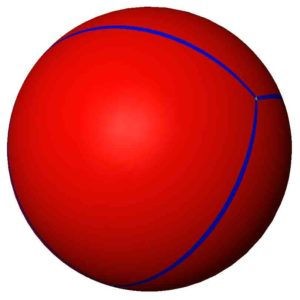The Tetrahedron in Metatron’s Cube: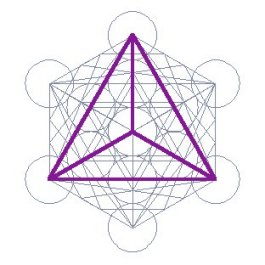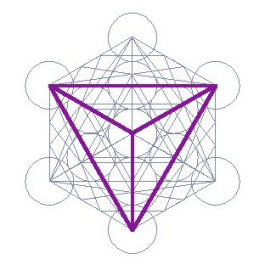The Sum of the angles of a tetrahedron = 720º = 1 x 2 x 3 x 4 x 5 x 6

This is because there are 4 equilateral triangles with 180º each.  180 x 4 = 720.

720 hertz = tone F# in music.Tetrahedral Projections

The tetrahedron has three 2-fold axes, passing through the midpoints of its edges.

It has four 3-fold axes, passing through one vertex and the center of the opposite face.

The following are some viewpoints that are commonly encountered:3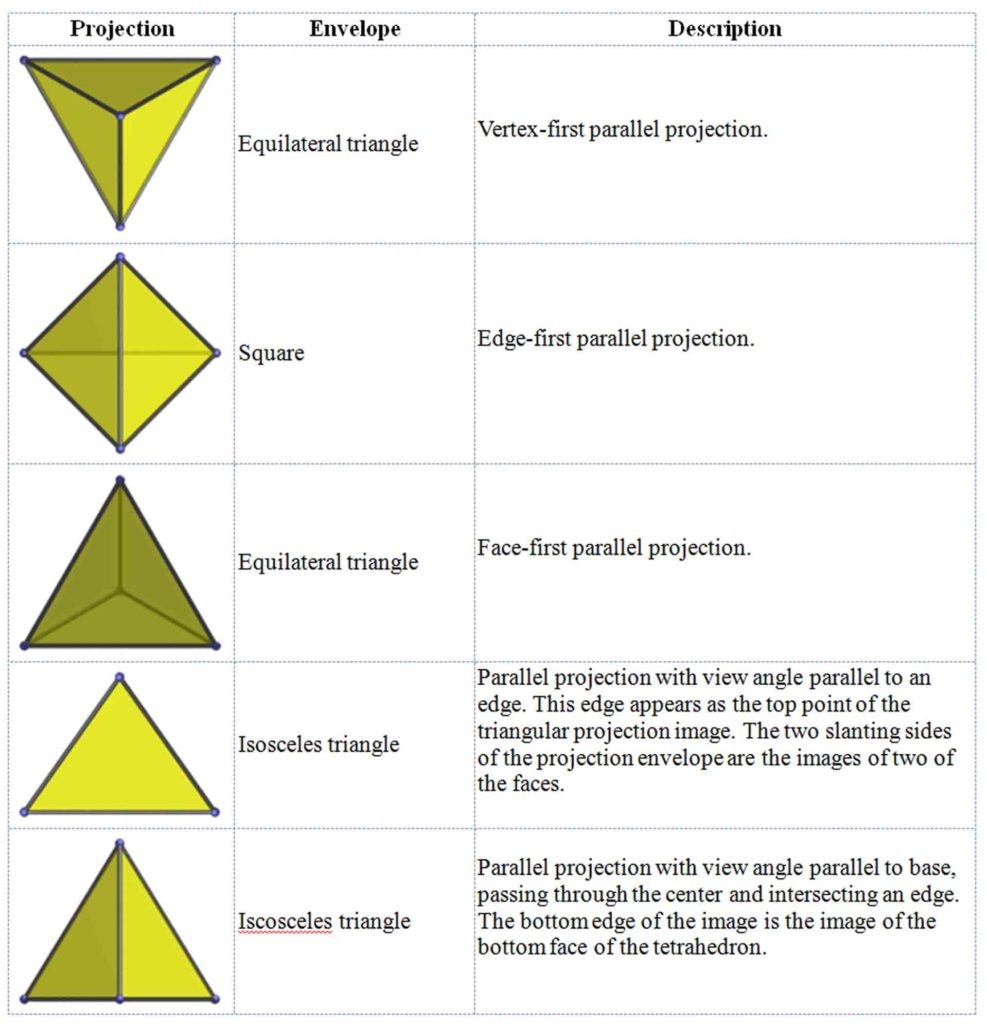In the tetrahedron the inradius (radius of the insphere) is 1/3 the circumradius (radius of the circumsphere).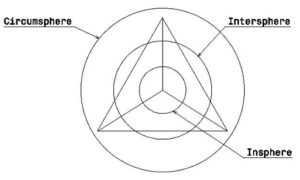The following image shows a tetrahedron with its insphere.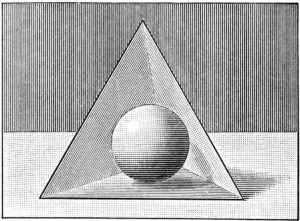Here we see a tetrahedron with its midsphere.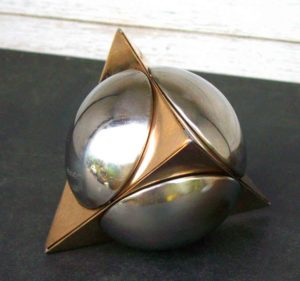And here we see a tetrahedron with its circumsphere.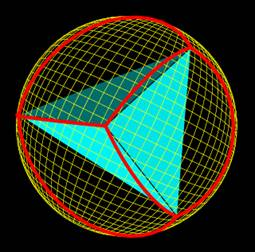Volume of a Tetrahedron

Volume = (s3√2)/12                        or                     V = (s3)/6√2

s = length of the side

Surface Area = √3s2

s = length of a side

Recall the Significance of the Number 720

72 = the number of years required for the equinoctial sun to complete a precessional shift of one degree along the ecliptic

The sum of the angles for all Platonic solids, Archimedean solids and Catalan solids are a factor of 72.

• 72 = The exterior angles of a regular pentagon
• 720 = sum of the angles of a tetrahedron
• 720 = sum of angles of a hexagon
• 720 x 9 = 6480 = sum of the angles of a dodecahedron
• 720 x 11 = 7920 = Diameter of the Earth in miles
• 720 x 12 = 8640; 8640 x 10 = 86400 = number of seconds in a day
• 720 x 12 = 8640 x 100 = 864000 = diameter of the Sun in miles
• 720 x 14 = 10080 = sum of angles of icosidodecahedron
• 720 x 29 = 20880 = sum of the angles of 4 Archimedean solids: rhombicosidodecahedron, snub dodecahedron, truncated icosahedron, and truncated dodecahedron

The Tetrahedron Used as Stability

As we saw, the tetrahedron is the most stable shape.  We see its use in:

• tripods
• three-legged stools
• campfires
• wheelbarrows
• tricycles – three wheels support the top point (the seat) for greatest stability
• insects walking – they walk on three legs at a time, alternating every other leg from one side to the other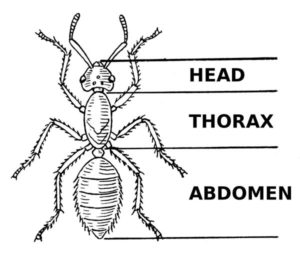• lotus position – stabilizes the body for the most stable and undisturbed position for the top point (the mind)The Tetrahedron in Physics – The Photon

We have covered this many times in Cosmic Core, yet it is worth repeating.  The shape of the photon has been found to be tetrahedral in nature.  This is yet another very important reason that the tetrahedron is delineated as the “master solid”.  We explain in Cosmic Core how all matter is composed of photons.  This idea that all is made of light is an ancient spiritual teaching that has been proven correct by modern science.Photons confine, loop and spin to form subatomic particles.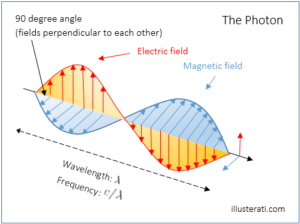These in turn loop and combine to form atoms.  Atoms combine to form molecules.  Molecules combine to form matter.  Always, at the heart of this process are the photons – or tetrahedral energy patterns of light.

The Tetrahedral Geometry of Subatomic Particle Interactions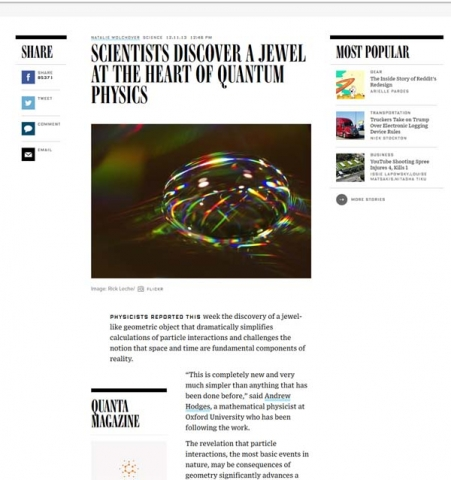The amplitudehedron is the geometry of subatomic particle interactions.  Every part is a tetrahedron.

“The amplitudehedron is a newly discovered mathematical object resembling a multifaceted jewel in higher dimensions.  Encoded in its volume are the most basic features of reality that can be calculated – the probabilities of outcomes of particle interactions.”4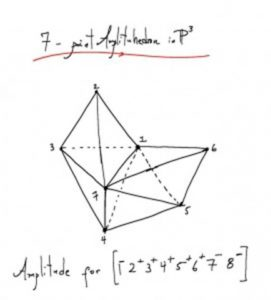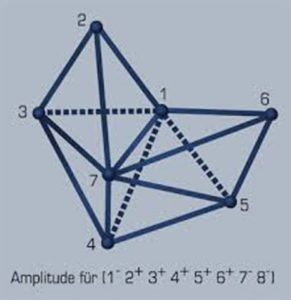The Amplitudehedron creates the universe in its image.  It is a shape that creates amplitudes that jiggle particle tracks in bubble chambers.

It shows the universe is an emanation of a single geometric form.  All is light.

The Amplitudehedron appears to be a star tetrahedron.  More precisely, ¼ of a star tetrahedron.

Notice below how the shape is one corner of the star tetrahedron (Merkaba/Stellated Octahedron).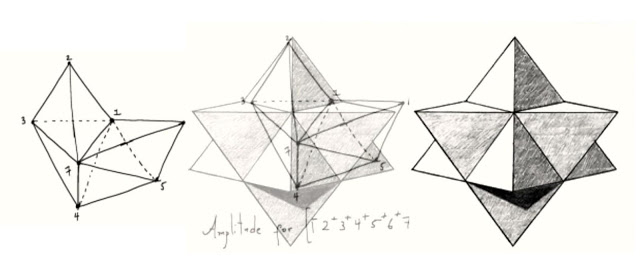Credit: David Wilcock

The Geometry of Light – The Photon

The core of the photons identity is geometric, just as the nature of all vibration in the Aether is geometric.

The photon is tetrahedral in nature.  This was posited by Max Planck, Buckminster Fuller, Rod Johnson, David Wilcock and Drunvalo Melchizadek, among others.

Free photons are two tetrahedra back to back with a conjoined base.  These fly through space in straight-line spirals at the speed of light and are not visible.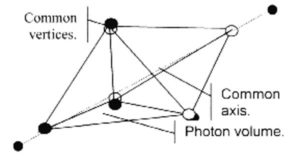Confined photons are a star tetrahedron – this is where the two tetrahedra slide together to form the star tetrahedron.  When this occurs the photons are visible.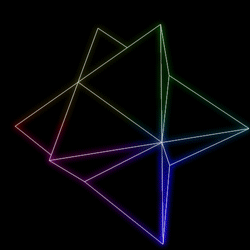In 2016 the shape of the photon was independently verified by Polish physicist Dr. Radoslaw Chrapkiewicz.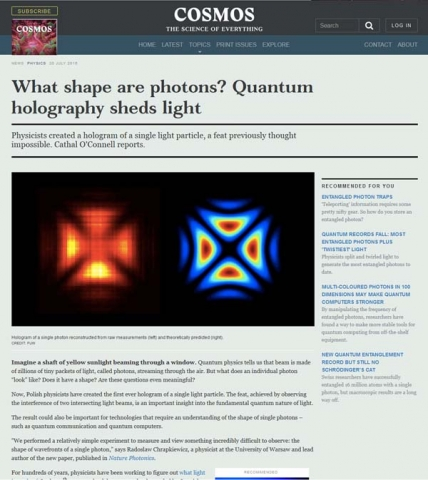They discovered the shape of the photon was a star tetrahedron looked at from its ‘cubic’ angle.  It looks exactly like a Maltese cross.

This shape was predicted by Schrodinger’s equation for the quantum wave function (pictured on right below.)

In the arms of the cross where the photons were in step, the image is bright.  Where they weren’t, we see darkness.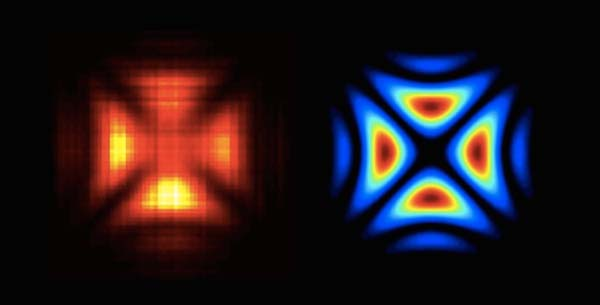Therefore, the photon is tetrahedral in nature and appears to be a star tetrahedron.

Remember, the vesica piscis is delineated by two equilateral triangles – in 3D they are tetrahedra.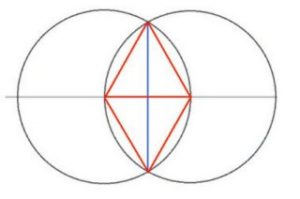The length and width of the vesica form a cross – the cross is the foundation of light.  One axis is electric, the other magnetic.  As they spiral around one another they create and carry electromagnetism.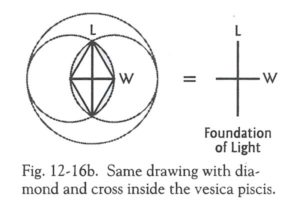The crossing of the tetrahedron, star tetrahedron and octahedron reflect the shape of the Maltese Cross above.

Note the 2-fold symmetry of the single tetrahedron as it appears as a cross.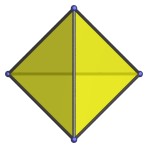Also note the 2-fold symmetry of the octahedron as it appears as a cross.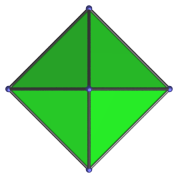Now note the ‘cubic’ angle of the star tetrahedron and how it appears as a cross.  Then remember that inside of every star tetrahedron is an octahedron.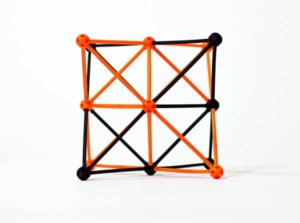The Tetrahedron in Chemistry

34 = 81 = number of stable elements in the periodic table

The tetrahedron occurs with great frequency in organic and inorganic chemistry.

There is tetrahedral molecular bonding: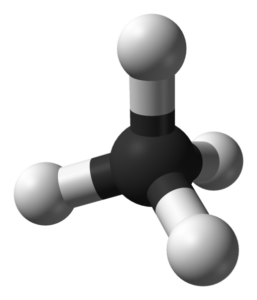Four bonds share one central atom.

There are no extra unshared electron pairs.

The Ideal Bond Angles = 60º or 109.47º (arccos(-1/3)

Remember, the angles in bonding patterns of molecules largely determine the qualities of the substance.

The tetrahedron frames the elements of:

• CH3F
• H2O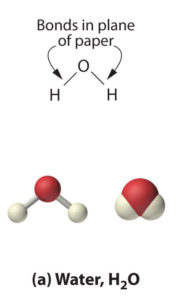• methane (CH4)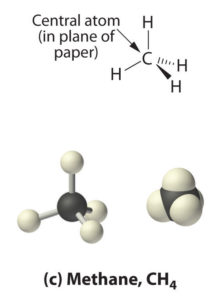• ethane (C2H6)
• ammonia (NH3)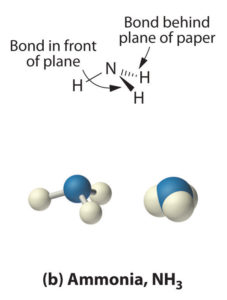• carbon tetrachloride
• the basis of amino acids – buildings blocks of life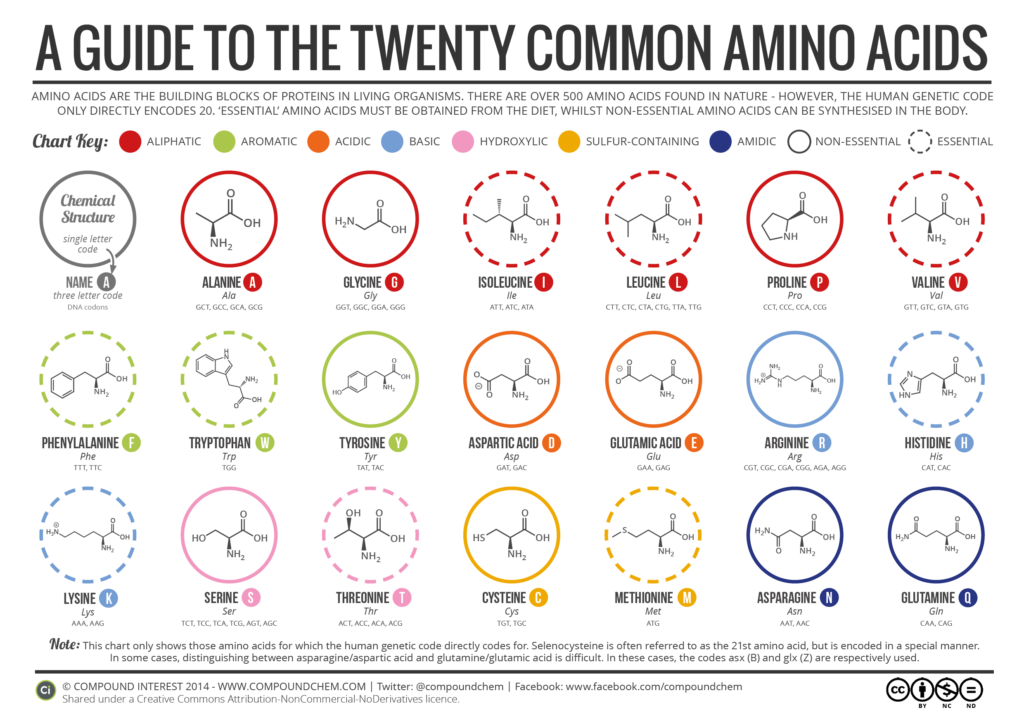• quartz – silicon dioxide (SiO2)
• Every silicon atom is at the center of a tetrahedron with oxygen atoms at its four corners.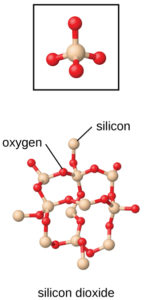• diamonds – structured as a tetrahedral network of only carbon atoms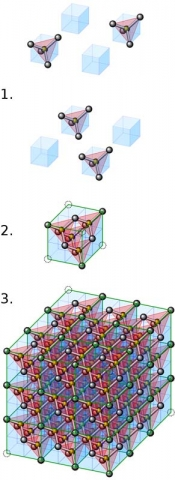• Each is at the center of a tetrahedron having four other carbon atoms at its corners.
• Diamonds are the hardest naturally occurring substance on Earth.
• They are best conductor of heat.
• Diamond cutters cut them along their tetrahedral faces.

The tetrahedron also shows up in tetrahedral crystal shape and family systems.

Some tetrahedral crystals include tetrahedrite, spinel, and magnetite.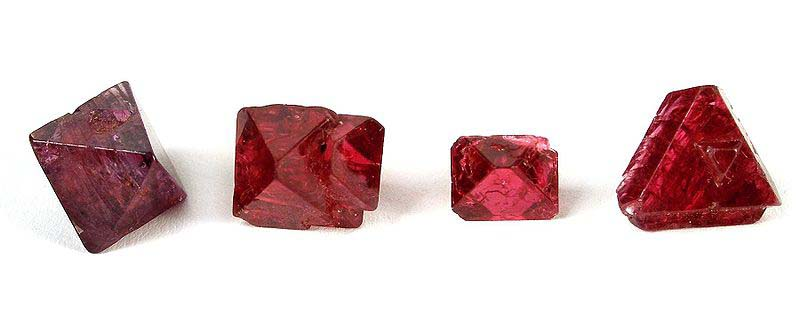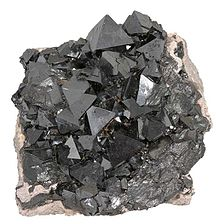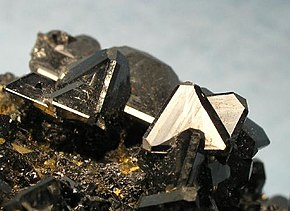Spinel, magnetite & tetrahedrite

The Tetrahedron & the Cube

A tetrahedron fits inside a cube.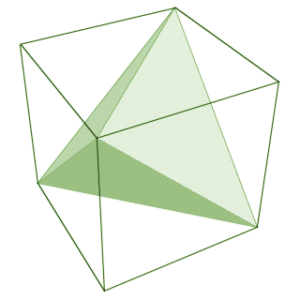If you draw a single diagonal line on all six faces of a cube so they join at the corners, you will have defined the edges of a tetrahedron.

The volume of the tetrahedron is 1/3 that of the cube.

The tetrahedron side length = √2 * cube edge length.

Recall that the diagonal of a square is √2 if the side length is 1.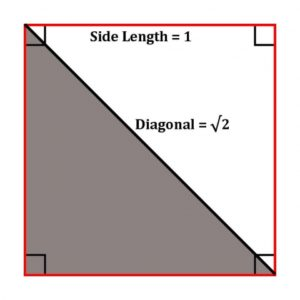You can see from the above image that the side of the tetrahedron is the diagonal of the square.  Hence, the side length of the tetrahedron will be √2 multiplied by the side length of the cube.

Here are two interlocking tetrahedra (star tetrahedron) in a cube: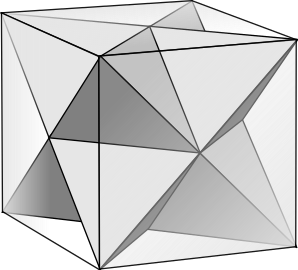Note the ‘cubic angle’ and ‘cross’ of the star tetrahedron.

The Tetrahedron, Dodecahedron and Icosahedron

A tetrahedron nests within the dodecahedron.  See below how the corners of the tetrahedron fall at vertex points of the dodecahedron.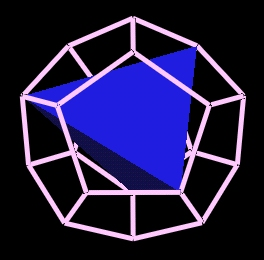Five full tetrahedra will fit inside a dodecahedron in this way.  This is called the Compound of Five Tetrahedra.

Compound of Five Tetrahedra

This compound polyhedra is one of the 58 stellations of the icosahedron.

It is also seen as a faceting of a regular dodecahedron.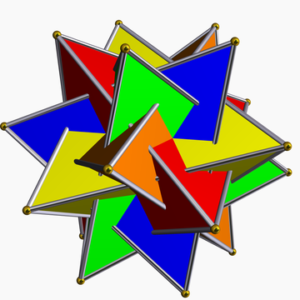From the above image you can see the five tetrahedra, each in a different color.

If you connect the outer vertexes you will draw the outline of a regular dodecahedron.

To see this, look at the top 5 points on this shape.  They are each of a different color.  Connecting those five points creates a pentagon.  You can then see how the dodecahedron forms around this compound.

“This compound is unusual, in that the dual figure is the enantiomorph of the original. If the faces are twisted to the right, then the vertices are twisted to the left. When we dualise, the faces dualise to right-twisted vertices and the vertices dualise to left-twisted faces, giving the chiral twin. Figures with this property are extremely rare.”5

Compound of Ten Tetrahedra

This compound can be seen as a different stellation of the icosahedron.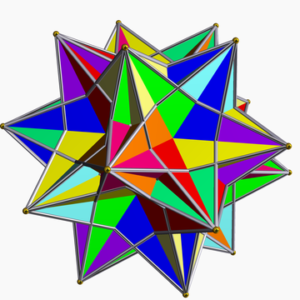Like the Five Tetrahedra compound, it also can be seen as a faceting of a regular dodecahedron.

Each of the ten tetrahedra is seen in a different color.  It is interesting to see how they naturally interweave.

It also shares the vertex arrangement of a regular dodecahedron, as described above.

It can also be made from the compound of five cubes by replacing each cube with a star tetrahedron (stella octangula) on the cube’s vertices.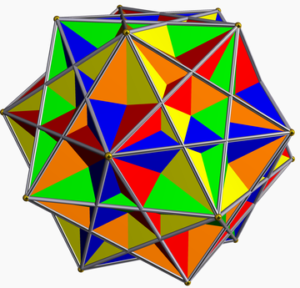These are just some of the many ways that tetrahedra, dodecahedra, icosahedra and cubes are interrelated.

The Tetrahedron & the Octahedron

The tetrahedron nests within an octahedron.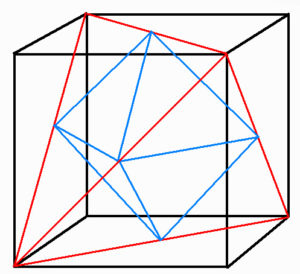The image above shows an octahedron nesting within a tetrahedron within a cube.

The 6 edge midpoints of the tetrahedron define the 6 vertices of the octahedron.

The octahedron halves the tetrahedron’s edges.

The octahedron halves the tetrahedron’s surface area & volume.

This embodies the octave 1:2.

Deeply truncating the tetrahedron (rectification) creates the octahedron.

This happens when the truncation planes move inward far enough to touch each other.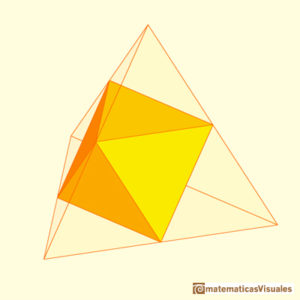The ‘degenerate truncation’ (or rectification) of a tetrahedron turns out to be the octahedron.

An octahedron also nests within a tetrahedron.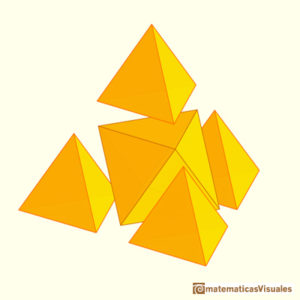And remember, stellating an octahedron gives you a star tetrahedron (stellated octahedron, stella octangula).

The Tetrahedron & the Chestahedron

Recall the Chestahedron, the only known seven-sided figure with all faces of equal area.  It was discovered by artist and geometrician Frank Chester.

The Chestahedron can be created in two distinct ways from a tetrahedron:

First way: Contractive

Place a tetrahedron inside a cube, and while keeping the boundaries of the cube inviolate, twist the tetrahedron so that one of its points moves along the diagonal of one square face to the opposite corner.

The tetrahedron, at this point, has changed into the octahedron.

There are actually 2 moments between the transformation of the tetrahedron into the octahedron that result in the Chestahedron.

The geometry of the Chestahedron is a geometry of motion (vortex motion).

The Chestahedron itself is merely a balanced moment of rest in a whole field of geometric activity that involves all of the Platonic forms.

Second way: Expansive

This begins with the tetrahedron, which is then unfolded like a flower with three petals.

See this in detail at: www.frankchester.com/2009/chestahedron-from-a-tetrahedron/.

The opening of the tetrahedron immediately creates the seven-sided form with the addition of the three kite-shaped faces.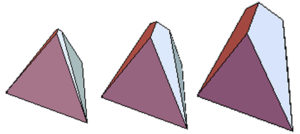It only remains to unfold the petals to the exact angle at which the area of the kite faces equals the area of the equilateral triangles at angle 94.83º.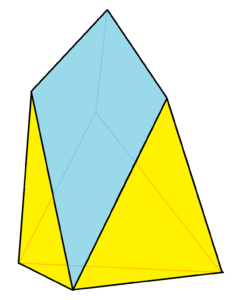This unfolding sequence, traced through time, can be taken further.

If the unfolding of the petals continues past the point at which the Chestahedron arises, a moment comes when the dihedral angle becomes 109.47°.

At this moment it takes the shape of a perfect octahedron with a tetrahedron on top that is exactly the size of the original tetrahedron.

This whole form itself is bounded by a tetrahedron that is exactly twice the size of the original.

The Tetrahedron in the Sphere

Recall from Article 30 that an equilateral triangle in a circle has many golden ratio proportions built in.

As a tetrahedron is composed of four equilateral triangles, when placed in a sphere, there will be many golden ratios.

See below in a 2D triangle: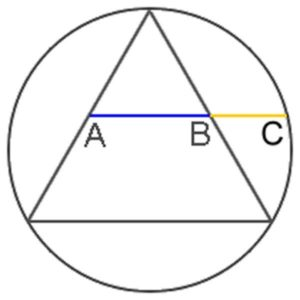The distance of AB = 1 and the distance of AC = φ (1.618…).

Or, BC = 1 and AB = φ (1.618…)

The Tetrahedron in a Sphere and the 19.47 Latitude

The tetrahedron in a sphere research originally came from Tom Bearden, a retired Lt. Colonel in the US Army.  It was later popularized by Richard C. Hoagland and David Wilcock.

If you place a tetrahedron in a sphere the 3 points of the base of the tetrahedron lie on the 19.47 latitude meridian of the circumsphere.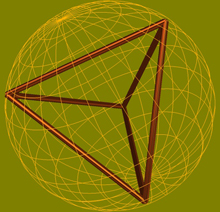If you transcribe a tetrahedron onto the sphere of a planet, the top point will be on the North Pole and the other three points will be at the approximate 19.5 degree South latitude.

Conversely, you could put an upside down tetrahedron and the three points will still be on the 19.5, but they will be in the North.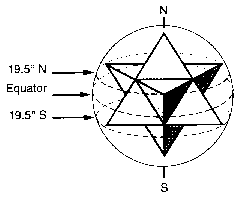These points are seen as major energetic points on Earth and in the solar system.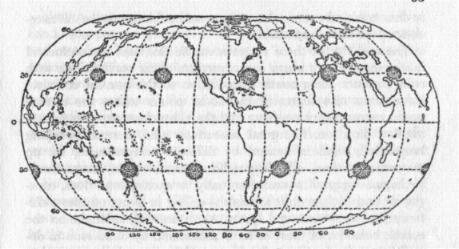Note that the degrees are not exactly at 19.47º in physical reality due to a wide range of naturally occurring variables and variations such as crust depth, potential changes over the eons (pole shifts, meteor collisions…etc.) and a wobble of the planetary and solar bodies that creates oscillations and imperfect rotations (the same wobble we see on Earth), as well as the fact that we are not dealing with perfect spheres but planets that slightly bulge in the center and have very irregular surface areas.

Also note that 33% of an hour has 19 minutes and 47 seconds (.33 x 60 = 19.8, .8 x 60 = 47).

Some examples discovered by Richard C. Hoagland & David Wilcock of the importance of the 19.47 latitude meridian include:

Earth:

Big Island of Hawaii

• Kilauea volcano – most continuously active volcano on Earth – 19.41º N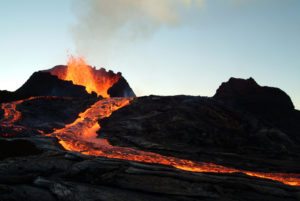• Mauna Kea – sacred giant shield volcano – 19.82º N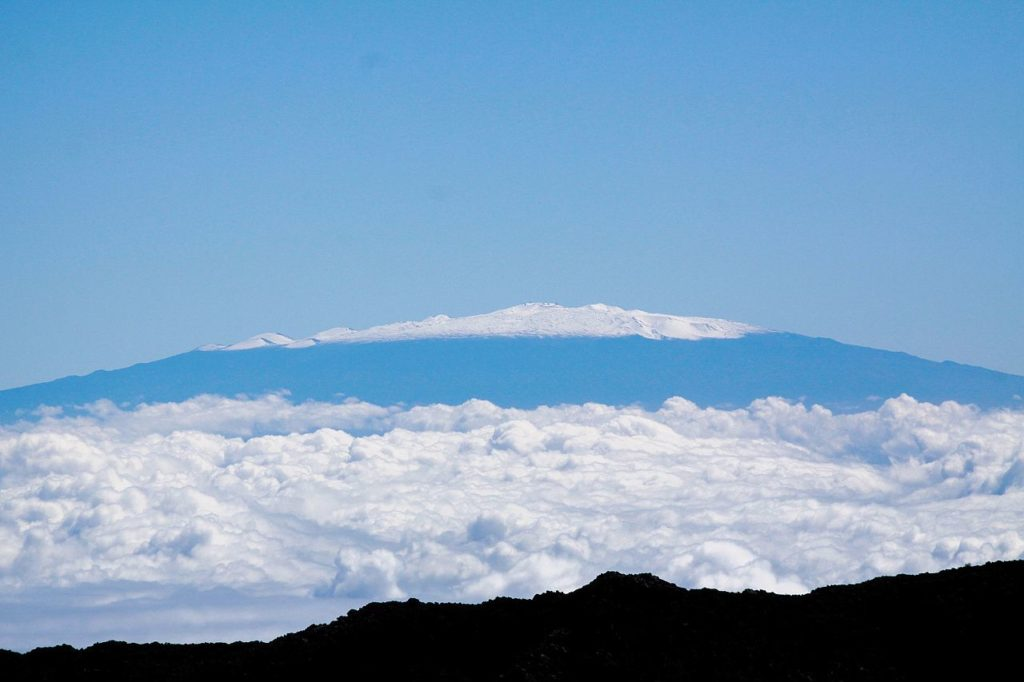• Mauna Loa – largest volcano on Earth (30 miles long, 60 miles wide) – 19.47ºN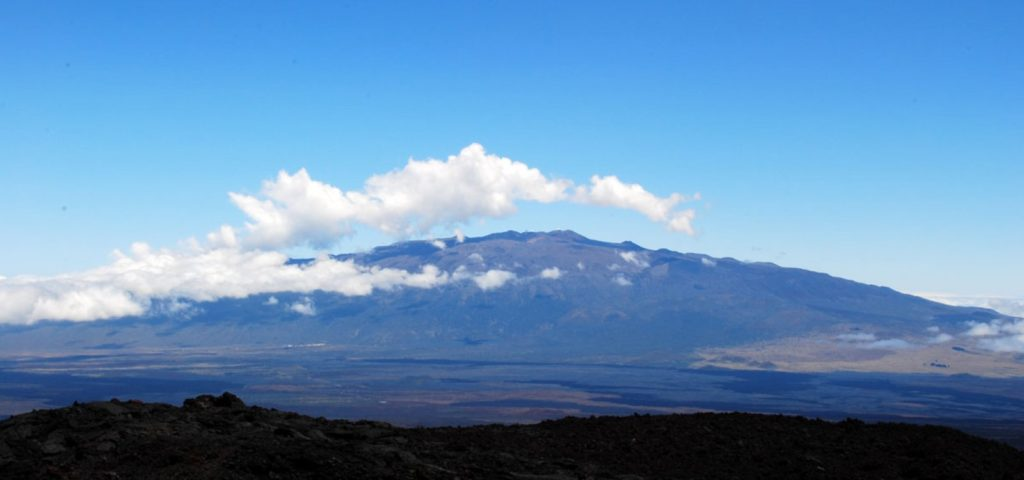Hurricanes most often spin off Africa from the 19.5º latitude.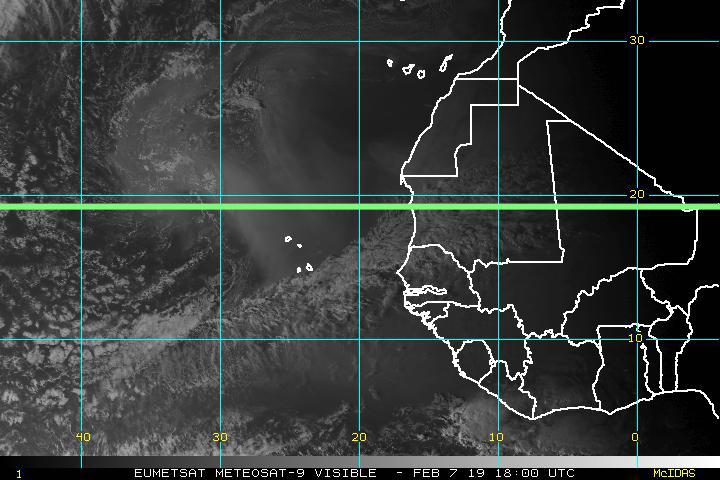Sacred monuments – Pyramids of the Sun & Moon (Mexico City)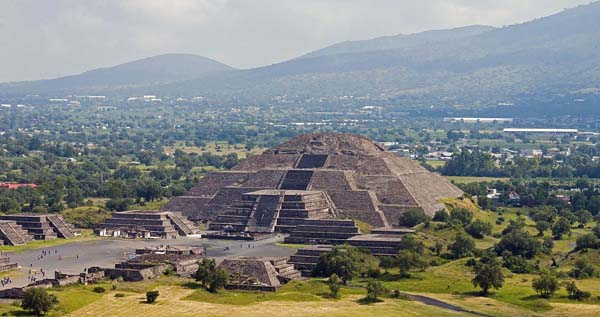Venus:

• Alta Regio 19.5º N; Beta Regio 25.0ºS – volcanic regions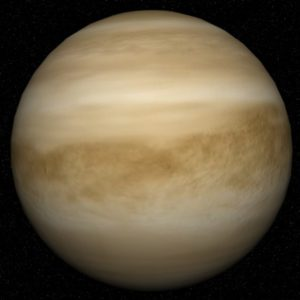• Strange highly-oxygenated plasma gas on Venus – charged atmosphere that recently showed up (Wilcock)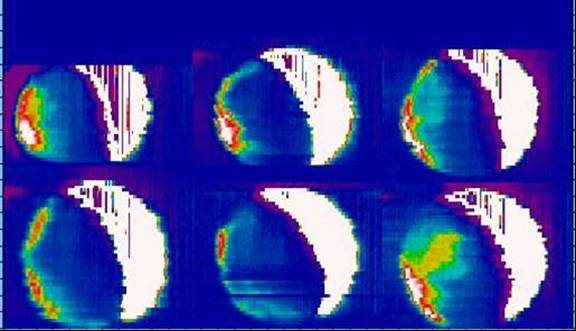• The plasma appears along the straight-line of the tetrahedron.
• Photo: Jeremy Bailey, ANU 2.3 cm CASPIR, Aus. Obs.
• This shows the tetrahedral geometry in Venus’s glow.  The white point in the lower left corner of Venus aligns with the 19.5 tetrahedral corner.

Mars:

• Olympus Mons – shield volcano – largest volcano in the solar system – 19.3º N
• It is 3x larger than Everest and looks like a giant version of Mauna Loa.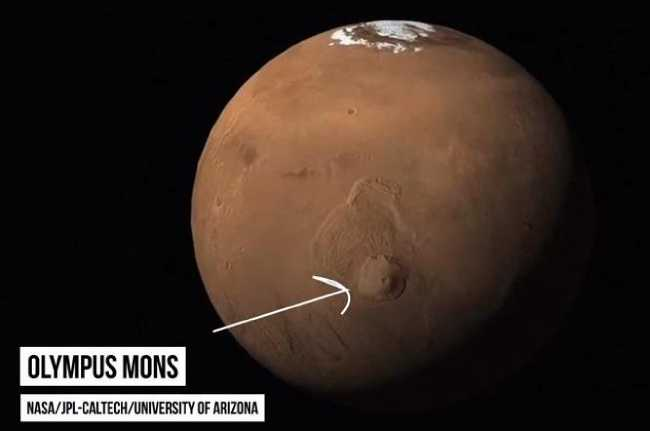• Mars Icecap melt from season to season (Wilcock) – a hexagonal pattern forms
• October 1996; January 1997; March 1997
• The geometry contains the boundary between cold and hot.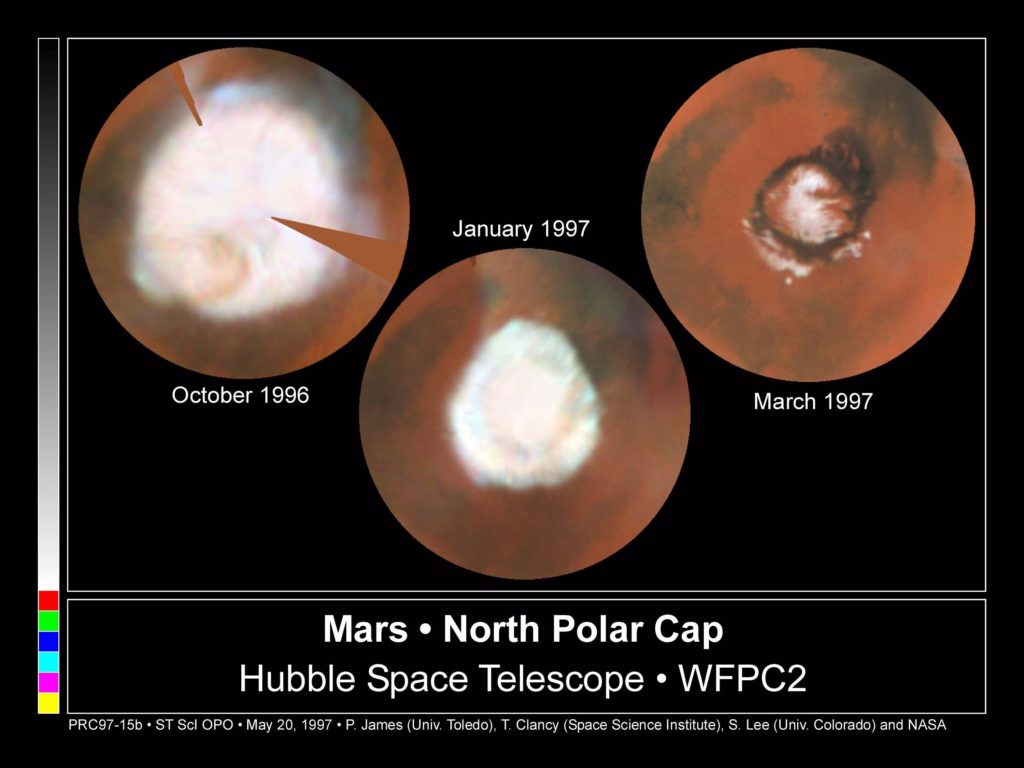North Polar Cap, Hubble Space Telescope WFPC2

Jupiter:

• Great Red spot – 22º S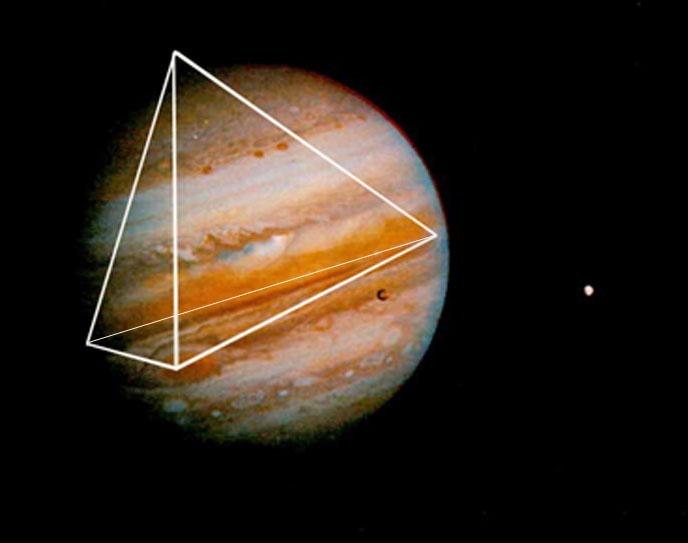• Bands of dark colors stay at 19.5 Lat. As the planet rotates the swirling vortexes at 19.5 spread out along the entire latitude.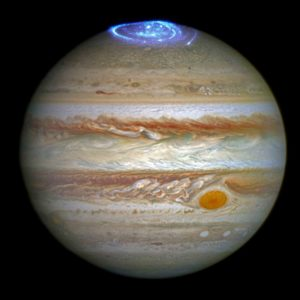• “Quasi-hexagonal” temperature drop in Jupiter’s northern polar region
• Looks like a pentagon, not a hexagon
• It is actually a dodecahedron – it precisely defines the boundary between hot and cold.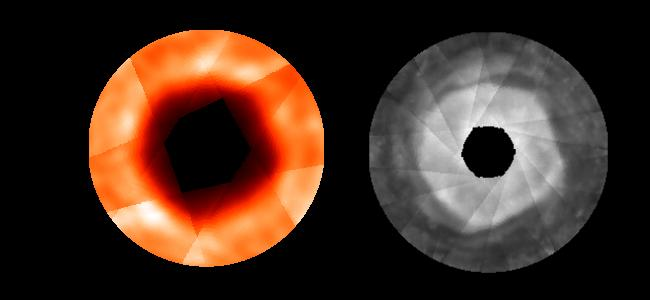Credit: JPL/NASA

Saturn:

• North/South Equatorial Belts – regions of storms observed from Earth – +/- 20º N/S
• These show the sharp differentiation between dark and light atmospheric conditions spreading out along the 19.5 latitude.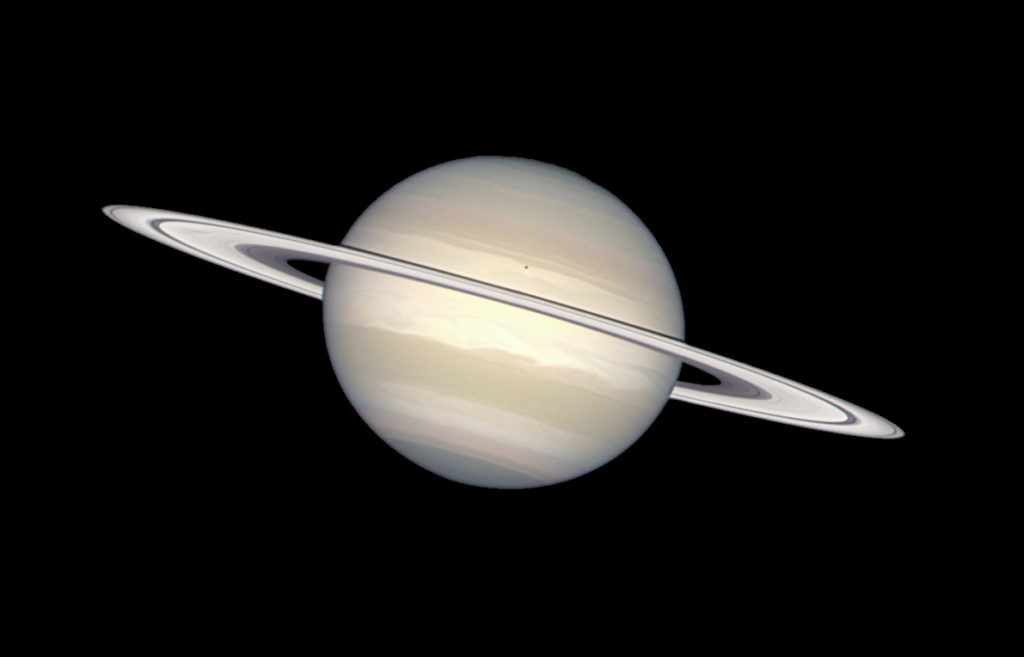Natural coloration of Saturn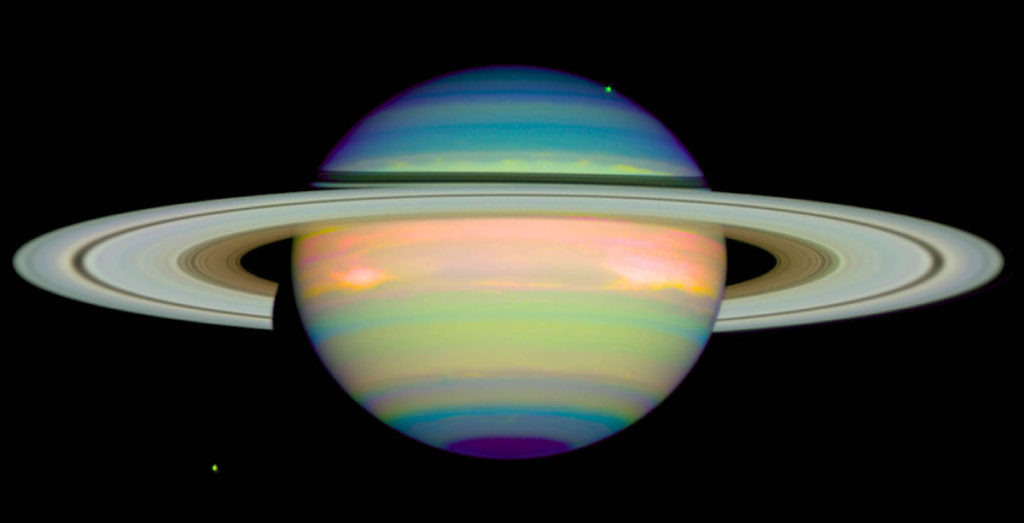Infrared Hubble image of Saturn

Uranus:

• Northern/Southern IR 1-2 K “Dip” – upwelling created by high-altitude clouds – +/- 20º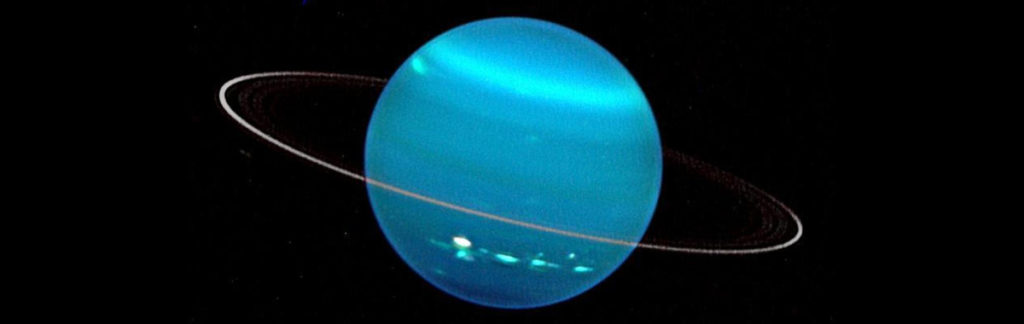Neptune:

• Great Dark spot – 22º S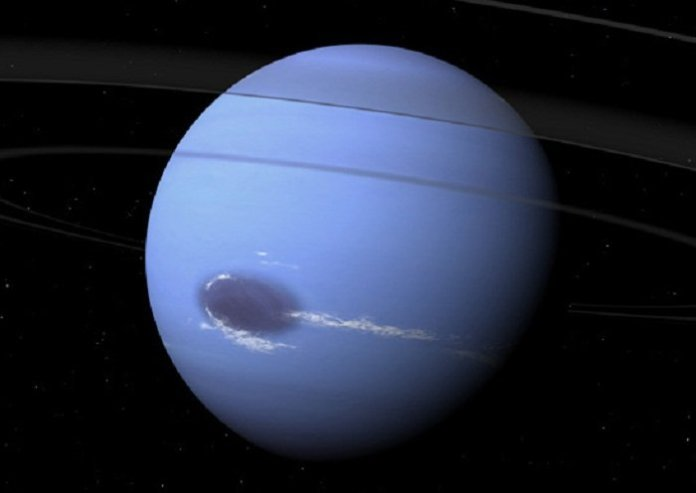Sun:

• Sunspot “peak” at 19.5º
• Sunspots tend to cluster at 19.5 N & S
• SOHO full disk continuum image – Aug 1999
• The sun spots move along as the sun rotates and so the sunspots trail along the 19.5 Lat.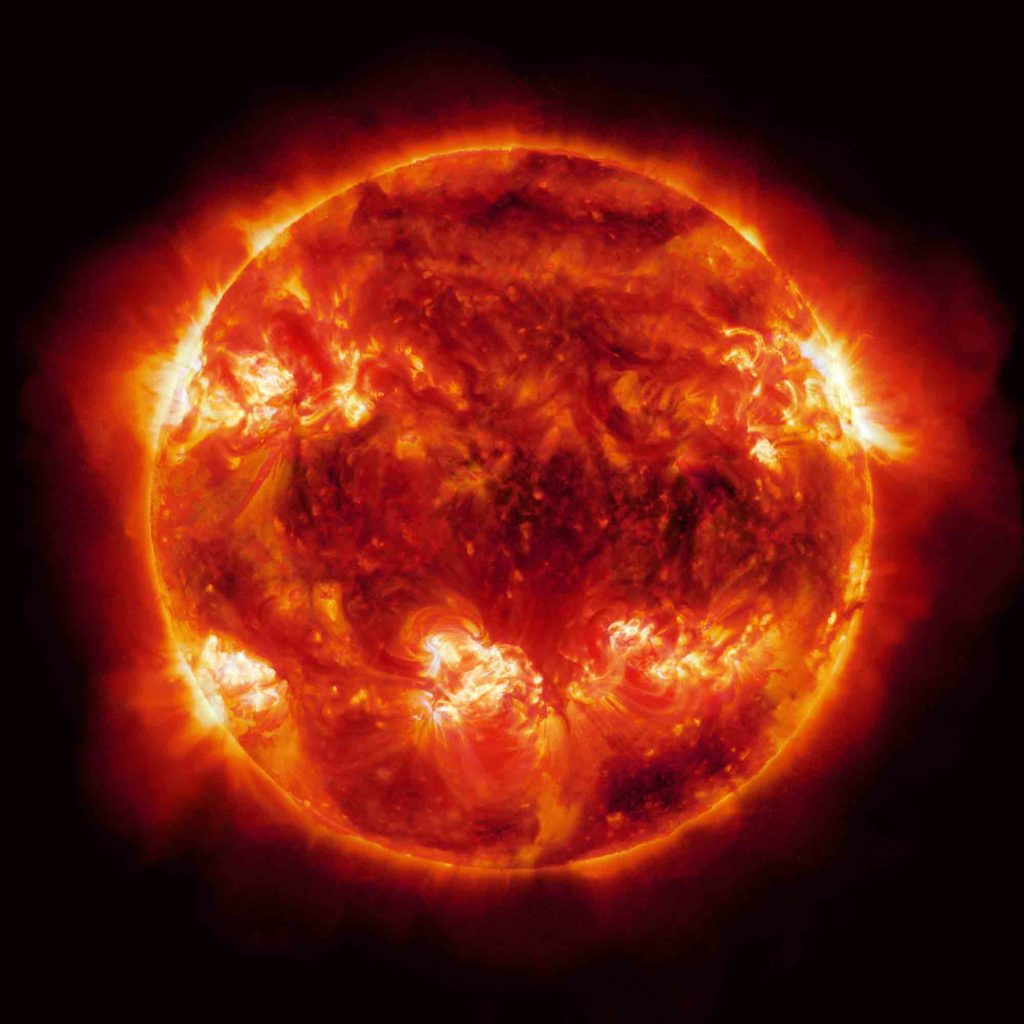Stellation of the Tetrahedron

There are no stellations of the cube or tetrahedron.  Recall: stellation refers to extending the facial planes past the edges of a given polyhedron until they intersect.

Here, however, is a beautiful design created by Ron Nelson at RNDmodels.6  There are 4 stellations here.  The shape sits like a tripod on three of the stellations.  The fourth reaches upward.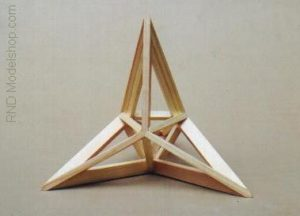Note that this shape is really just an upside down tetrahedron created in a beautiful, artistic way.  It is not a ‘true’ stellation.  It does show how tetrahedra are inside tetrahedra.

Truncations of the Tetrahedron

When a tetrahedron is uniformly truncated, it forms the truncated tetrahedron, an Archimedean solid.  This means all 4 vertices of a tetrahedron are truncated at one third of the original edge length.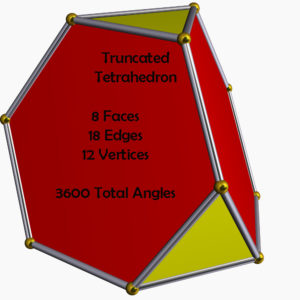It has:

• 4 regular hexagonal faces
• 4 equilateral triangle faces
• 12 vertices
• 18 edges (of two types)

The truncated tetrahedron has an angle sum of 3600º.

A = 7√3a2

V = (23√2a3)/12

Orthogonal Projection of the Truncated Tetrahedron

Here are some commonly encountered views of the truncated tetrahedron.6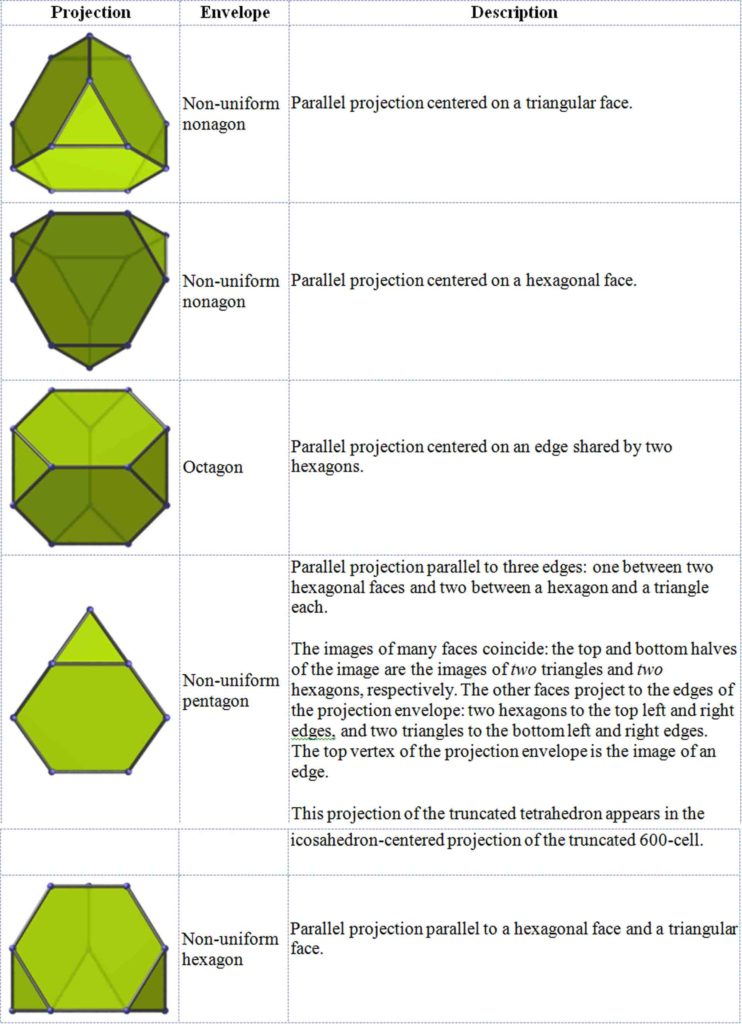Net of the Truncated TetrahedronSpherical Truncated Tetrahedron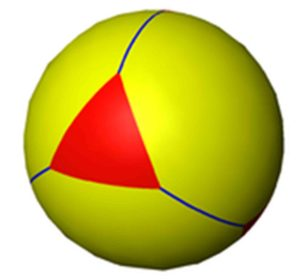Truncated Tetrahedron in Rotation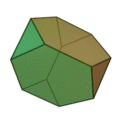Variations on Truncation of the Tetrahedron

You can have a very shallow truncation of the tetrahedron as seen here:

Four tiny tetrahedra are removed from each vertex.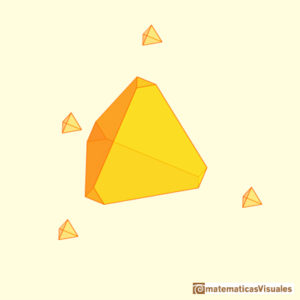Or you can have an extreme truncation of the tetrahedron as seen below.

This is the rectification of a tetrahedron and it produces the octahedron.This shows how intimately the tetrahedron is related to the octahedron.

The Compound of two Truncated Tetrahedra

This is a uniform polyhedron compound composed of two truncated tetrahedra, formed by truncating each of the tetrahedra in the stellated octahedron (star tetrahedron).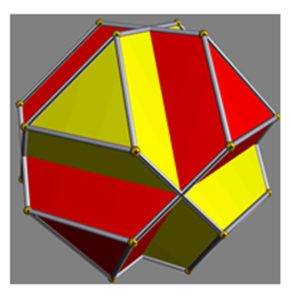Triakis Tetrahedron – 2160º

The triakis tetrahedron is the dual of the truncated tetrahedron.  It is a Catalan solid.

It can be seen as a tetrahedron with triangular pyramids added to each face.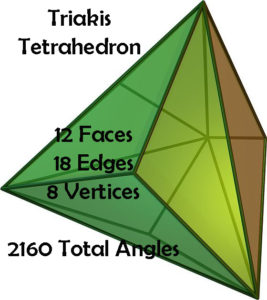It has:

• 12 Faces
• 18 Edges
• 8 Vertices

The faces look as follows: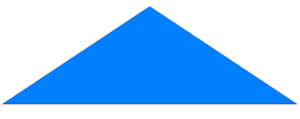Each Face is an isosceles triangle.

The triakis tetrahedron angle sum is 2160º.

Here is a rotating model: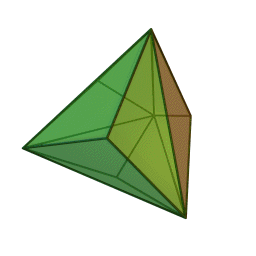Here is the Net: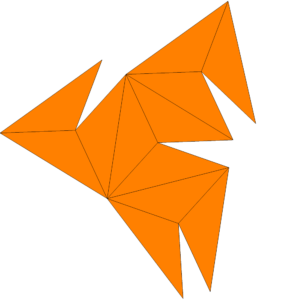1. http://www.matematicasvisuales.com/english/html/geometry/planenets/tetrahedronnet.html
2. Edmondson, Amy, A Fuller Explanation: The Synergetic Geometry of R. Buckminster Fuller, Burkhauser Boston, 1987
3. http://eusebeia.dyndns.org/4d/tetrahedron
4. Wolchover, Natalie, Scientists Discover a Jewel at the Heart of Quantum Physics, 12/11/13, https://www.wired.com/2013/12/amplituhedron-jewel-quantum-physics/
5. https://en.wikipedia.org/wiki/Compound_of_five_tetrahedra
6. https://rndmodels.deviantart.com/
7. http://eusebeia.dyndns.org/4d/trunctet